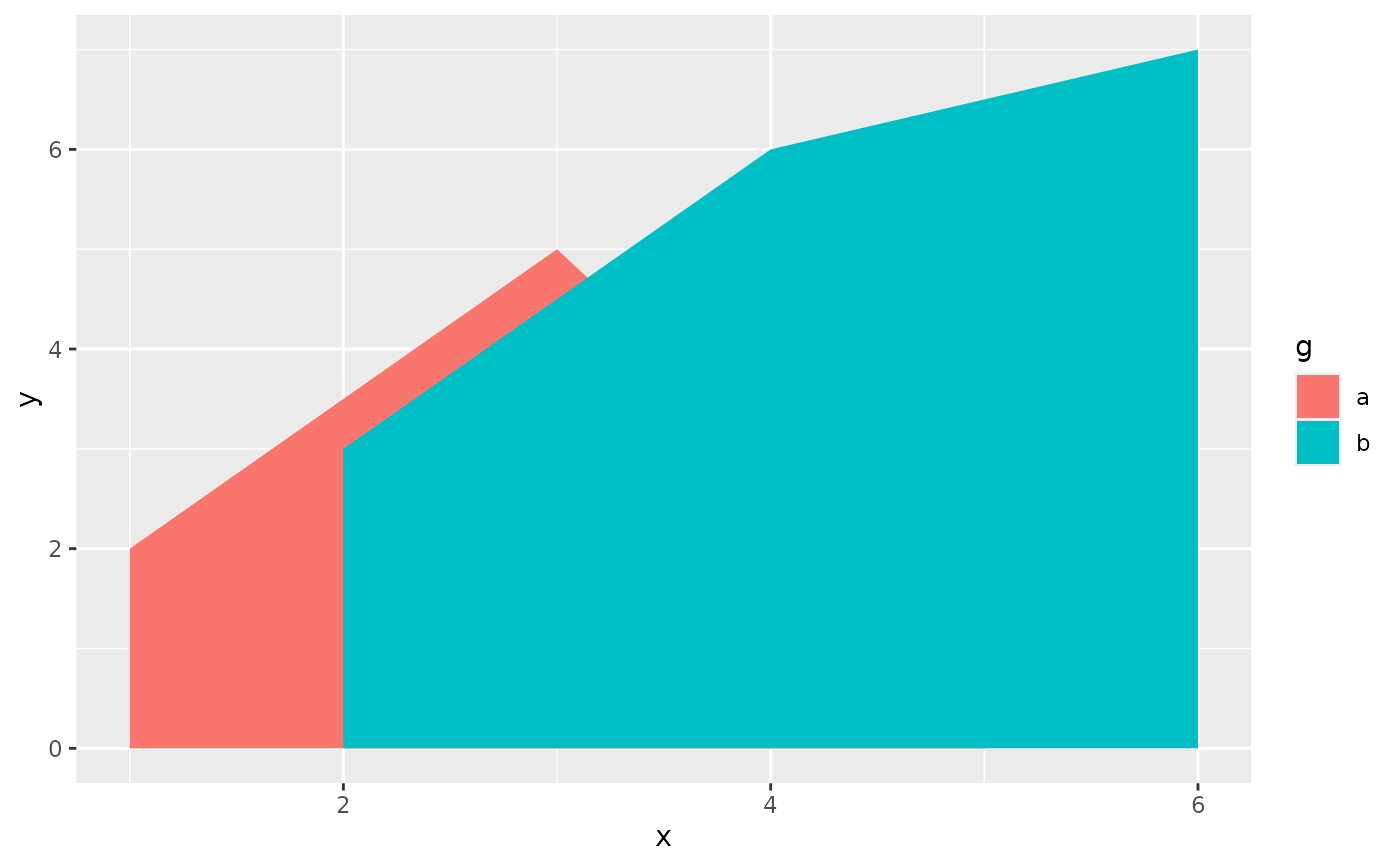For each x value, geom_ribbon() displays a y interval defined by ymin and ymax. geom_area() is a special case of geom_ribbon(), where the ymin is fixed to 0 and y is used instead of ymax.

## Usage

geom_ribbon(
mapping = NULL,
data = NULL,
stat = "identity",
position = "identity",
...,
na.rm = FALSE,
orientation = NA,
show.legend = NA,
inherit.aes = TRUE,
outline.type = "both"
)

geom_area(
mapping = NULL,
data = NULL,
stat = "align",
position = "stack",
na.rm = FALSE,
orientation = NA,
show.legend = NA,
inherit.aes = TRUE,
...,
outline.type = "upper"
)

stat_align(
mapping = NULL,
data = NULL,
geom = "area",
position = "identity",
...,
na.rm = FALSE,
show.legend = NA,
inherit.aes = TRUE
)

## Arguments

mapping

Set of aesthetic mappings created by aes(). If specified and inherit.aes = TRUE (the default), it is combined with the default mapping at the top level of the plot. You must supply mapping if there is no plot mapping.

data

The data to be displayed in this layer. There are three options:

If NULL, the default, the data is inherited from the plot data as specified in the call to ggplot().

A data.frame, or other object, will override the plot data. All objects will be fortified to produce a data frame. See fortify() for which variables will be created.

A function will be called with a single argument, the plot data. The return value must be a data.frame, and will be used as the layer data. A function can be created from a formula (e.g. ~ head(.x, 10)).

stat

The statistical transformation to use on the data for this layer, either as a ggproto Geom subclass or as a string naming the stat stripped of the stat_ prefix (e.g. "count" rather than "stat_count")

position

Position adjustment, either as a string naming the adjustment (e.g. "jitter" to use position_jitter), or the result of a call to a position adjustment function. Use the latter if you need to change the settings of the adjustment.

...

Other arguments passed on to layer(). These are often aesthetics, used to set an aesthetic to a fixed value, like colour = "red" or size = 3. They may also be parameters to the paired geom/stat.

na.rm

If FALSE, the default, missing values are removed with a warning. If TRUE, missing values are silently removed.

orientation

The orientation of the layer. The default (NA) automatically determines the orientation from the aesthetic mapping. In the rare event that this fails it can be given explicitly by setting orientation to either "x" or "y". See the Orientation section for more detail.

show.legend

logical. Should this layer be included in the legends? NA, the default, includes if any aesthetics are mapped. FALSE never includes, and TRUE always includes. It can also be a named logical vector to finely select the aesthetics to display.

inherit.aes

If FALSE, overrides the default aesthetics, rather than combining with them. This is most useful for helper functions that define both data and aesthetics and shouldn't inherit behaviour from the default plot specification, e.g. borders().

outline.type

Type of the outline of the area; "both" draws both the upper and lower lines, "upper"/"lower" draws the respective lines only. "full" draws a closed polygon around the area.

geom

The geometric object to use to display the data, either as a ggproto Geom subclass or as a string naming the geom stripped of the geom_ prefix (e.g. "point" rather than "geom_point")

## Details

An area plot is the continuous analogue of a stacked bar chart (see geom_bar()), and can be used to show how composition of the whole varies over the range of x. Choosing the order in which different components is stacked is very important, as it becomes increasing hard to see the individual pattern as you move up the stack. See position_stack() for the details of stacking algorithm. To facilitate stacking, the default stat = "align" interpolates groups to a common set of x-coordinates. To turn off this interpolation, stat = "identity" can be used instead.

## Orientation

This geom treats each axis differently and, thus, can thus have two orientations. Often the orientation is easy to deduce from a combination of the given mappings and the types of positional scales in use. Thus, ggplot2 will by default try to guess which orientation the layer should have. Under rare circumstances, the orientation is ambiguous and guessing may fail. In that case the orientation can be specified directly using the orientation parameter, which can be either "x" or "y". The value gives the axis that the geom should run along, "x" being the default orientation you would expect for the geom.

## Aesthetics

geom_ribbon() understands the following aesthetics (required aesthetics are in bold):

• x or y

• ymin or xmin

• ymax or xmax

• alpha

• colour

• fill

• group

• linetype

• linewidth

Learn more about setting these aesthetics in vignette("ggplot2-specs").

geom_bar() for discrete intervals (bars), geom_linerange() for discrete intervals (lines), geom_polygon() for general polygons

## Examples

# Generate data
huron <- data.frame(year = 1875:1972, level = as.vector(LakeHuron))
h <- ggplot(huron, aes(year))

h + geom_ribbon(aes(ymin=0, ymax=level))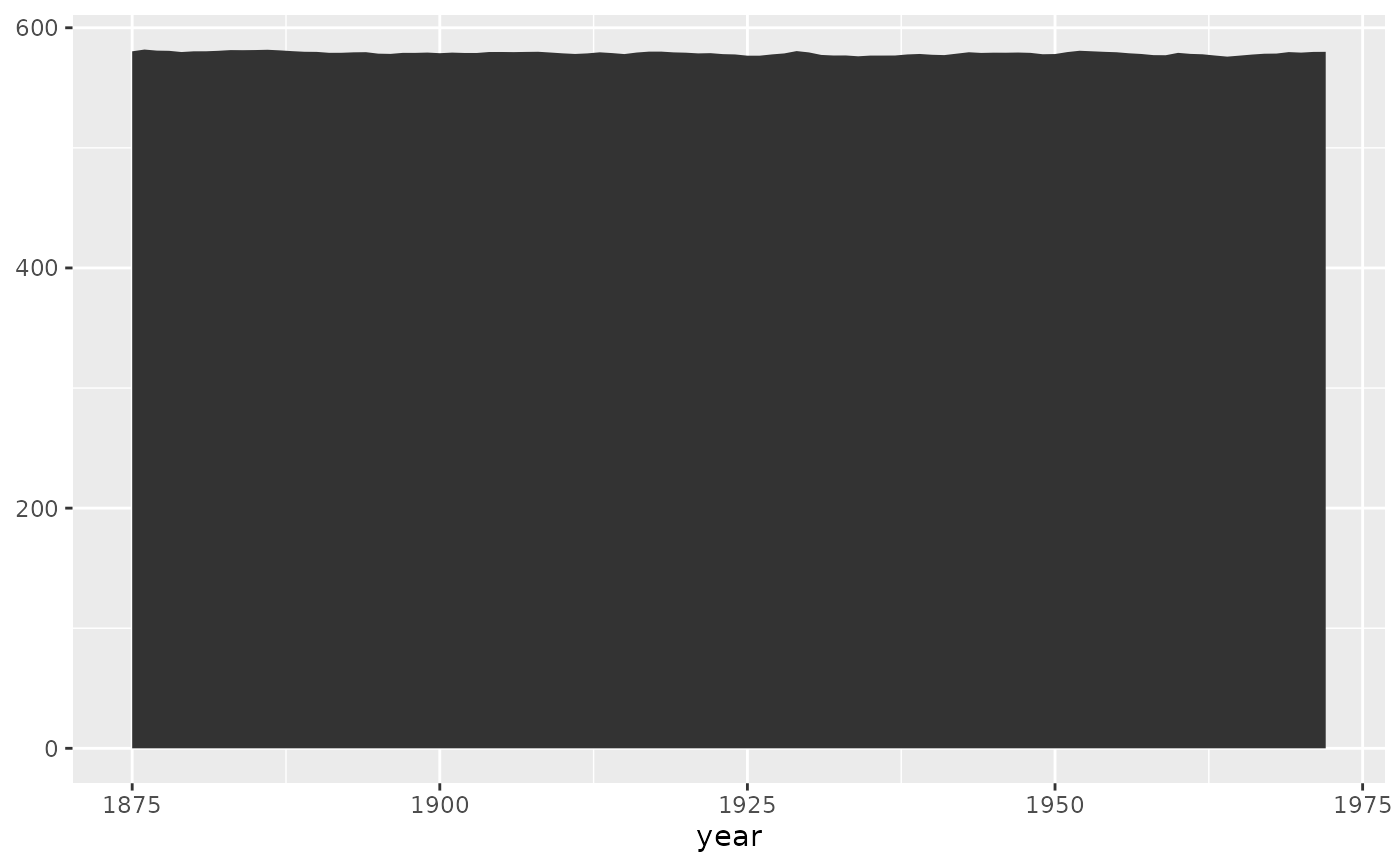h + geom_area(aes(y = level))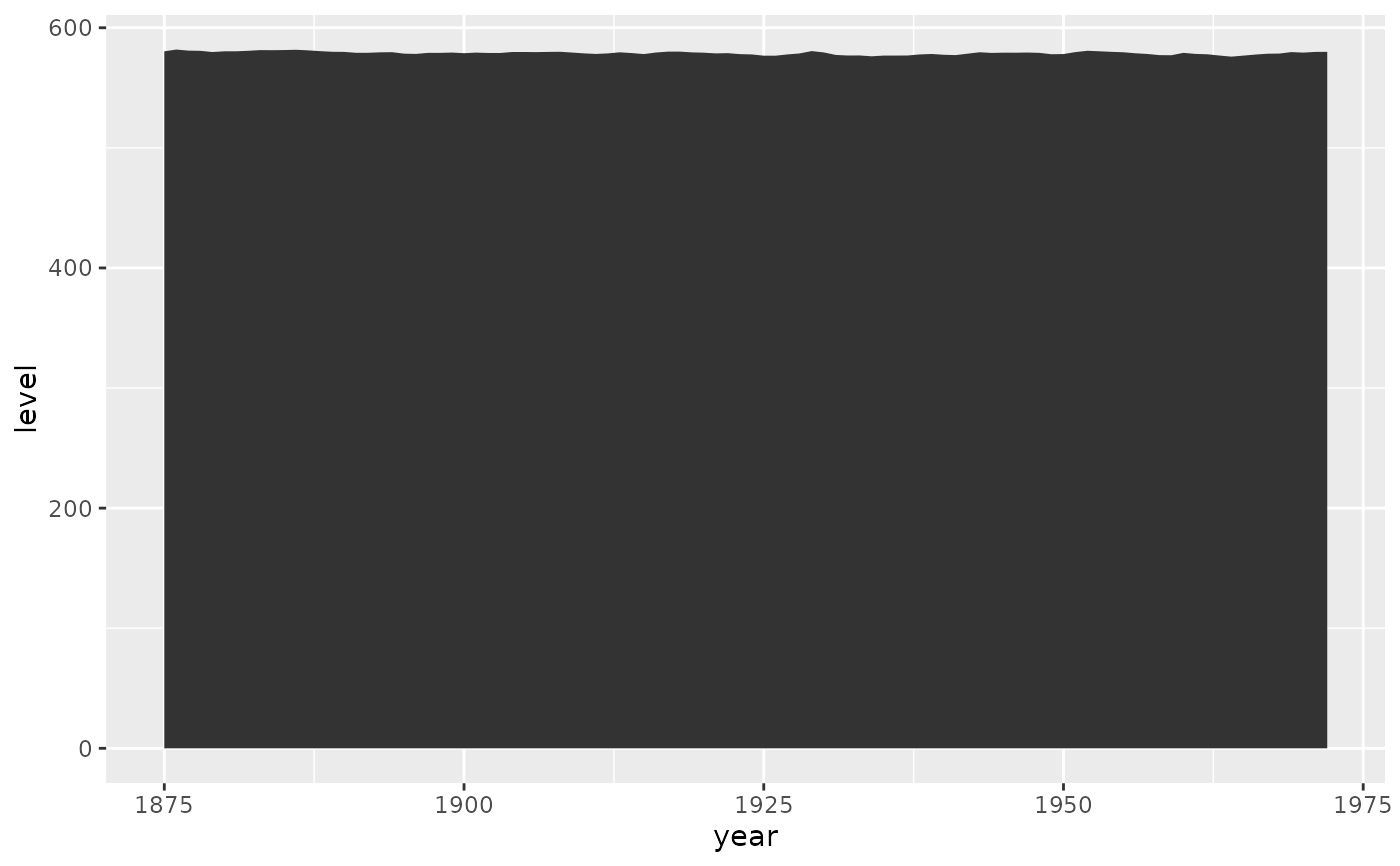# Orientation cannot be deduced by mapping, so must be given explicitly for
# flipped orientation
h + geom_area(aes(x = level, y = year), orientation = "y")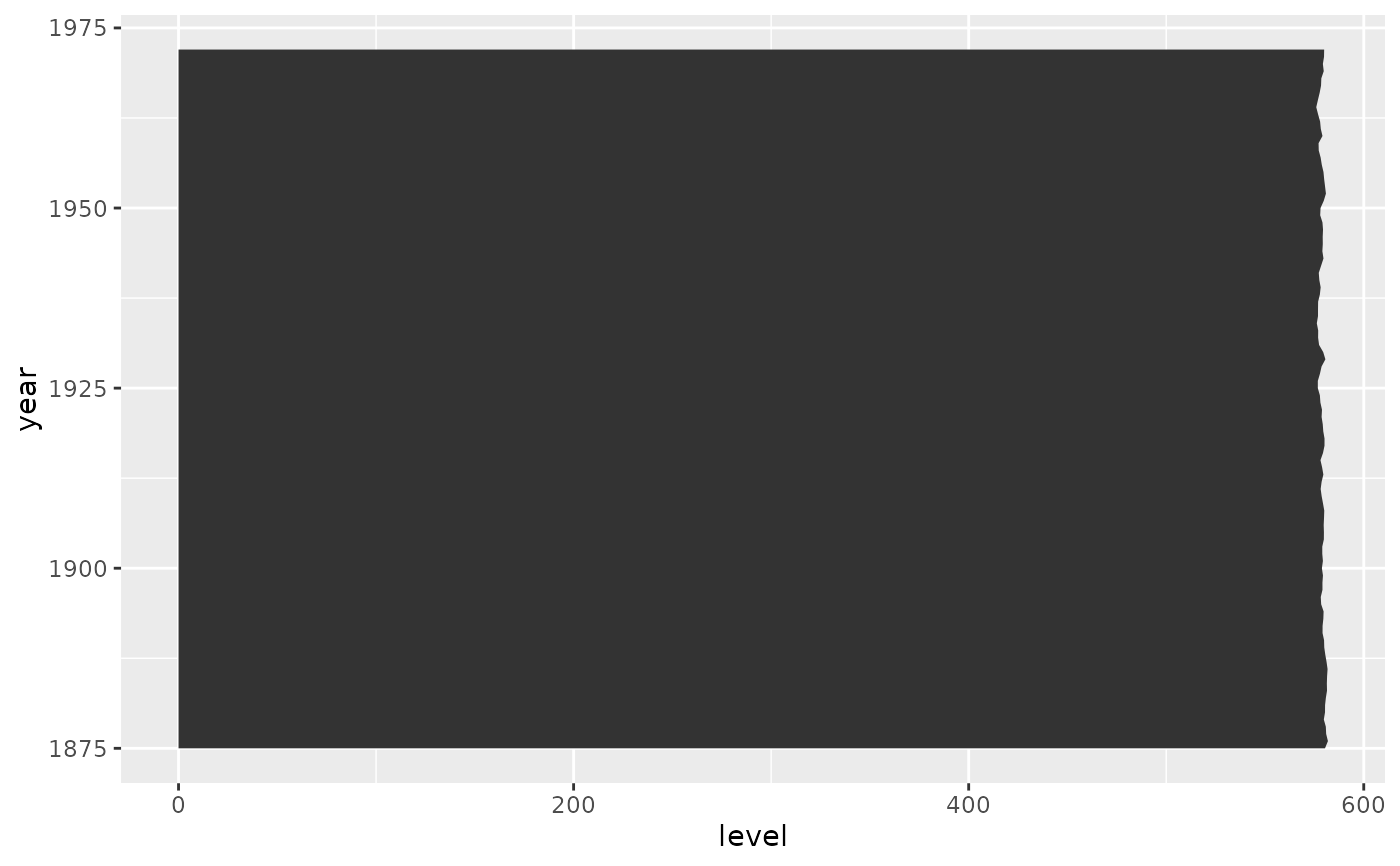h +
geom_ribbon(aes(ymin = level - 1, ymax = level + 1), fill = "grey70") +
geom_line(aes(y = level))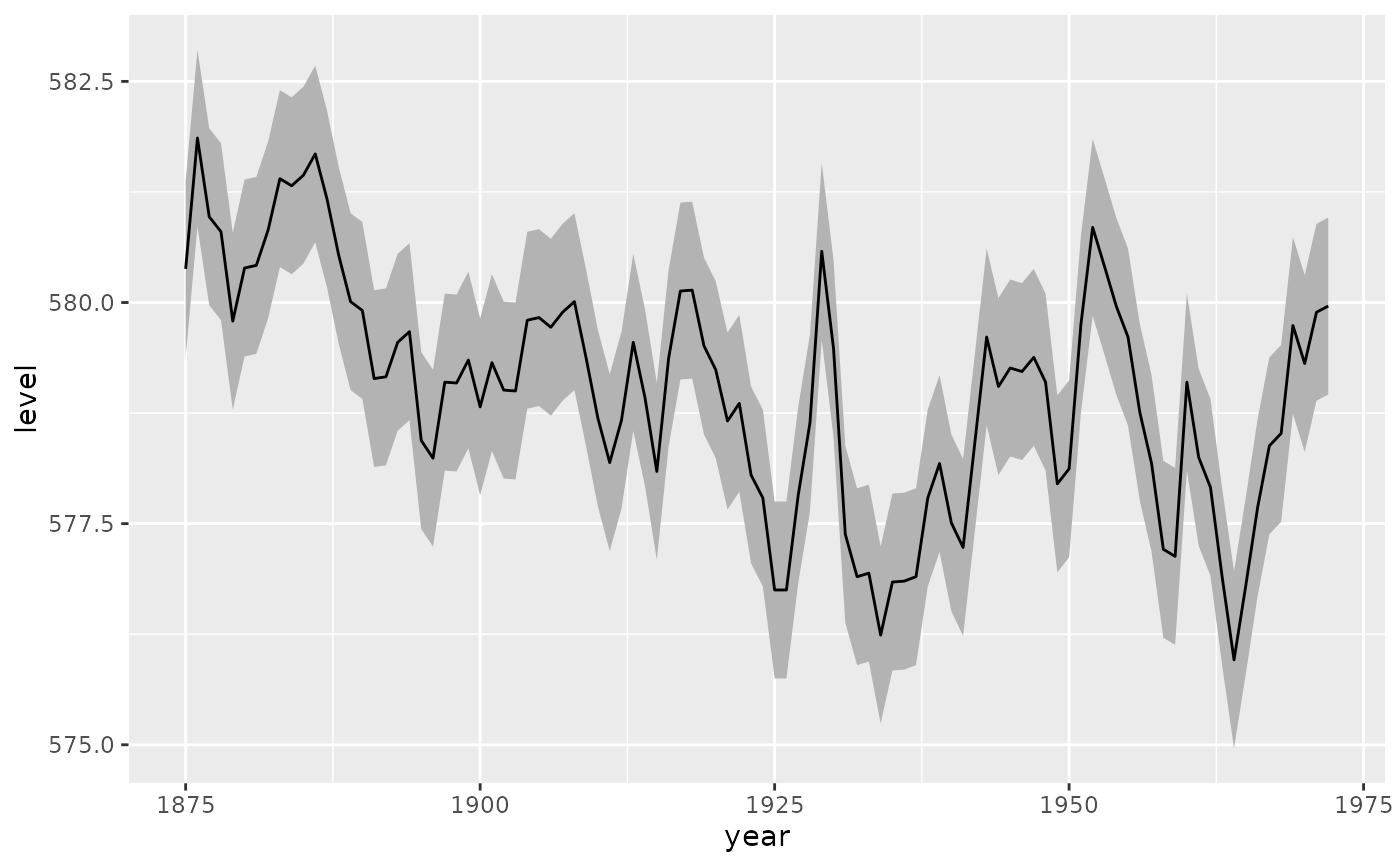# The underlying stat_align() takes care of unaligned data points
df <- data.frame(
g = c("a", "a", "a", "b", "b", "b"),
x = c(1, 3, 5, 2, 4, 6),
y = c(2, 5, 1, 3, 6, 7)
)
a <- ggplot(df, aes(x, y, fill = g)) +
geom_area()

# Two groups have points on different X values.
a + geom_point(size = 8) + facet_grid(g ~ .)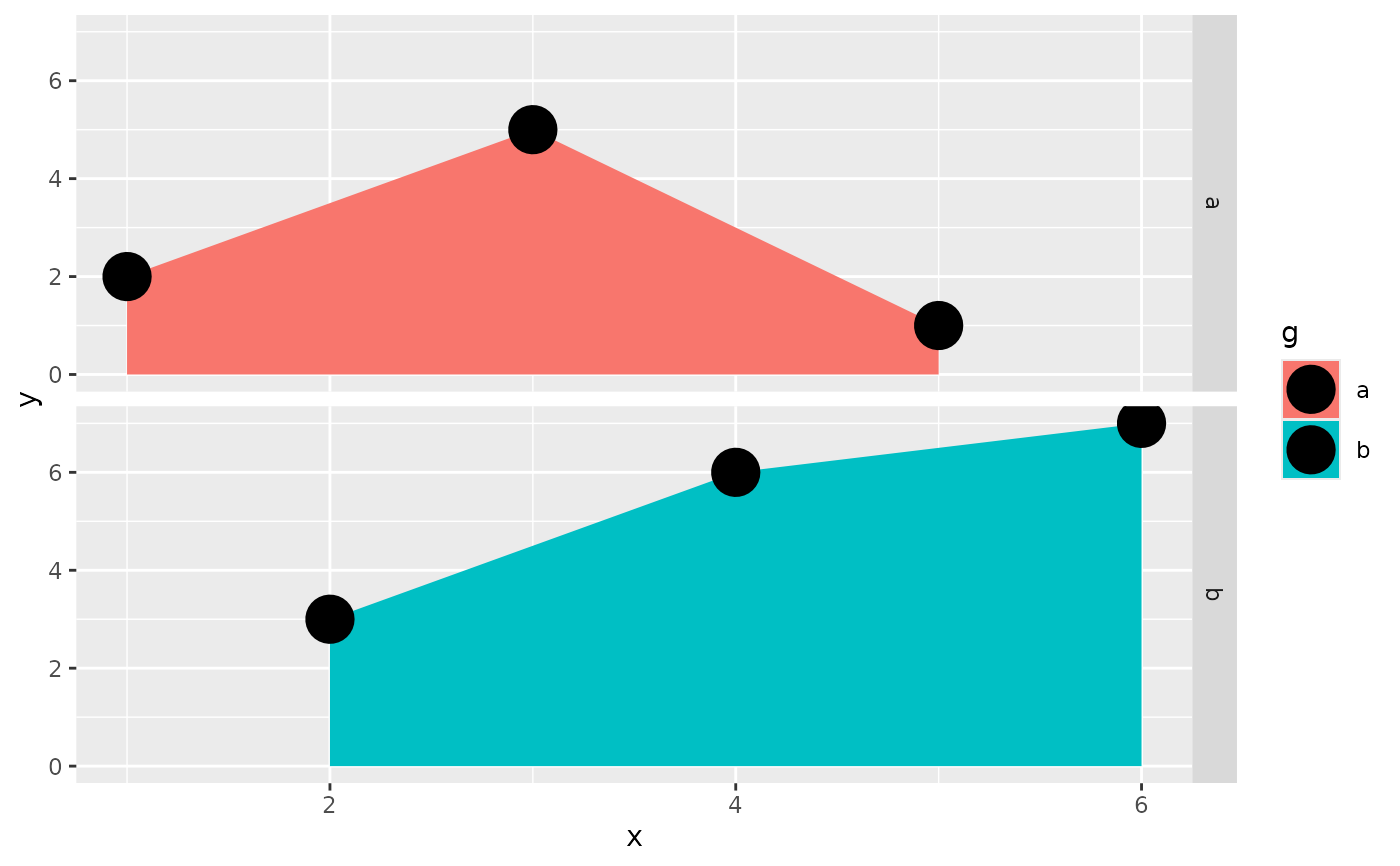# stat_align() interpolates and aligns the value so that the areas can stack
# properly.
a + geom_point(stat = "align", position = "stack", size = 8)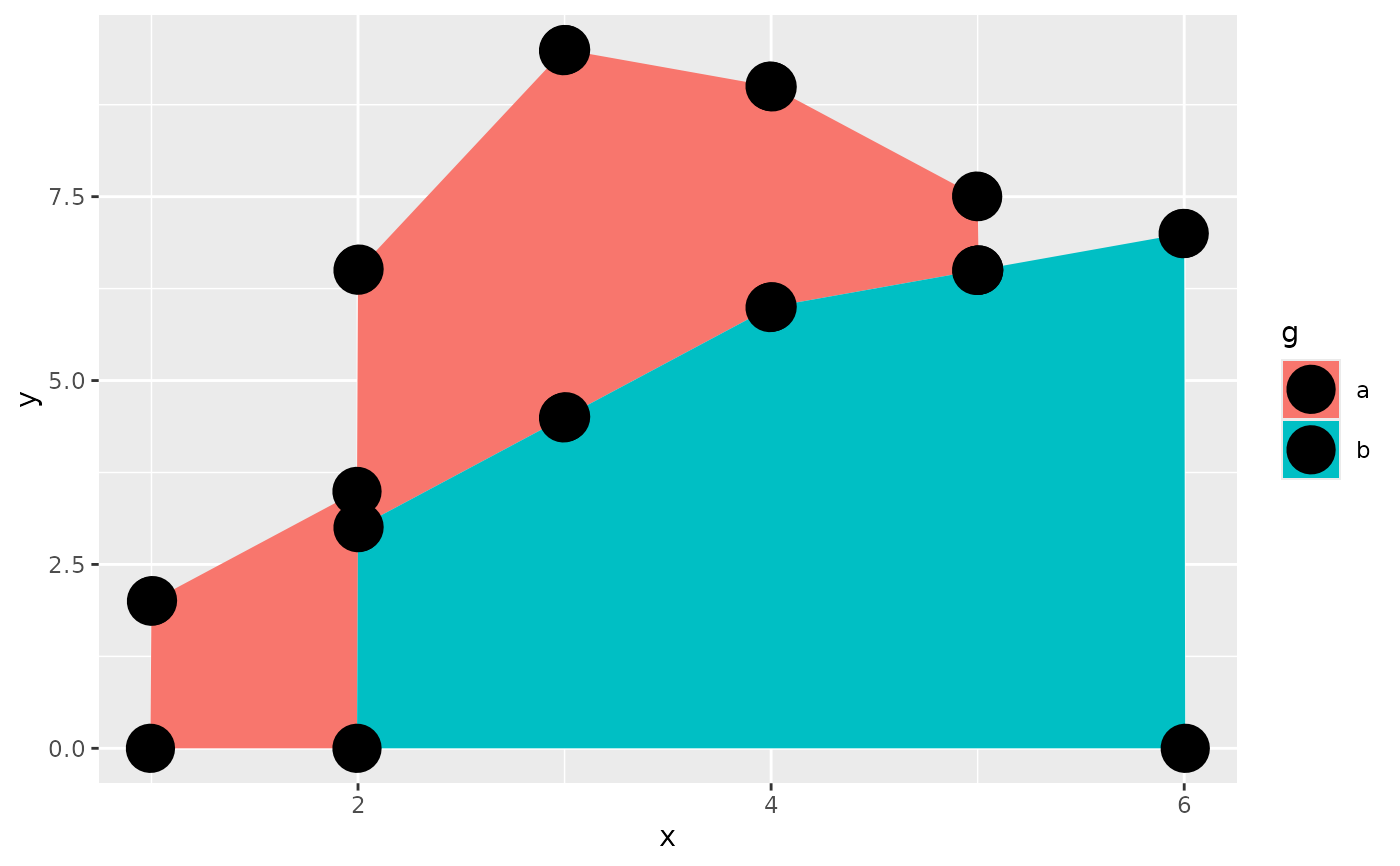# To turn off the alignment, the stat can be set to "identity"
ggplot(df, aes(x, y, fill = g)) +
geom_area(stat = "identity")